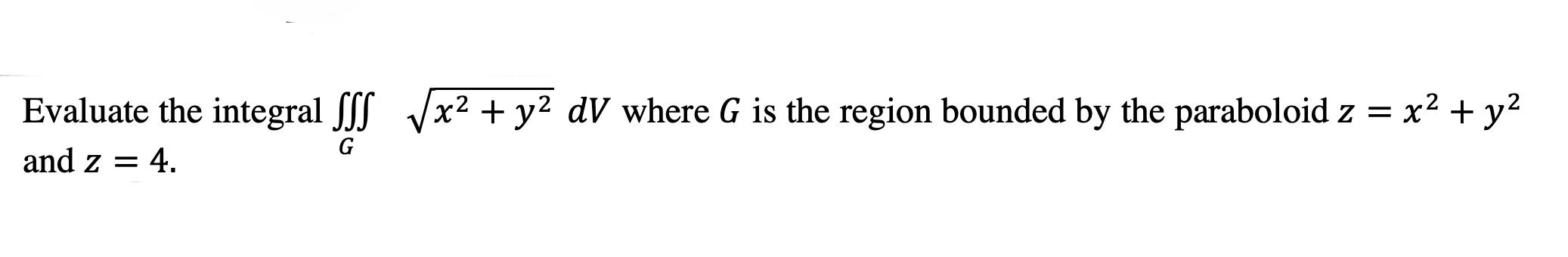### Create an Account

Already have account?

### Forgot Your Password ?

Home / Questions / Evaluate the integral SSS Vx2 + y2 dV where G is the region bounded by the paraboloid z = ...

# Evaluate the integral SSS Vx2 + y2 dV where G is the region bounded by the paraboloid z = x2 + y2 and z = 4. G

Evaluate the integral SSS Vx2 + y2 dV where G is the region bounded by the paraboloid z = x2 + y2 and z = 4. GMay 07 2021 View more View Less

#### Answer (Solved)Subscribe To Get Solution